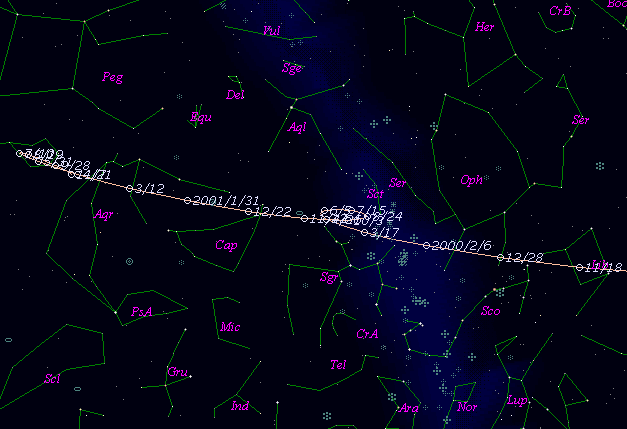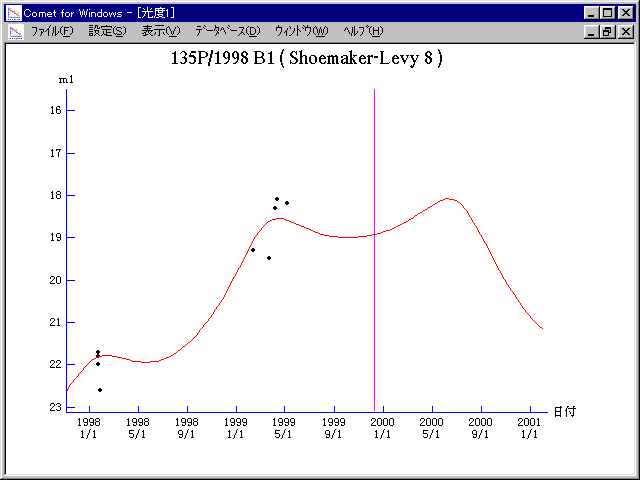# \$B%7%e!<%a!<%+!<(B-\$B%l%t%#Bh#8WB@1(B

135P/Shoemaker-Levy 8 (1999)###\$B%W%m%U%#!<%k(B

 \$BId9f(B 135P/1998 B1 \$B8!=PF|(B 1998\$BG/(B 1\$B7n(B22\$BF|(B \$B8!=P8wEY(B 21.8\$BEy(B \$B8!=P C. W. Hergenrother (Smithsonian Astrophysical Observatory)

###\$B50F;MWAG(B

```   The following improved orbital elements by Kenji Muraoka, are
from 86 observations 1992 to 1999, perturbations by 9 Plantes,
Moon and 5 minor planets were taken into account.
The mean residual is +/- 0.82 arc seconds.

Epoch  =  1999 Dec.  8.0  TT       JDT = 2451520.5
T  =  1999 Dec. 10.51715       +/- 0.00068 (m.e.) TT
Peri. =   22.58759                +/- 0.00025
Node  =  213.31746                +/- 0.00019   (2000.0)
Incl. =    6.05042                +/- 0.00003
q  =    2.7211912              +/- 0.0000020 AU
e  =    0.2894580              +/- 0.0000004
a  =    3.8297403              +/- 0.0000010 AU
n  =    0.13150737             +/- 0.00000005
P  =    7.495                  +/- 0.0000028  years
```

###\$B@1?^(B###\$B8wEYJQ2=(B

```        m1 = 8.5 + 5 log\$B&\$(B + 17.5 log r
```##### \$B50F;MWAG\$OB<2,7r<#;a\$N7W;;\$K\$h\$k\$b\$N\$G\$9!#(B \$B@1?^\$O(B StellaNavigator Ver.2.0 for Windows (\$B%"%9%H%m%"!<%D(B \$BJTCx(B / \$B%"%9%-!<=PHG6I4)(B) \$B\$G:n@.\$7\$?\$b\$N\$G\$9!#(B \$B8wEY%0%i%U\$O(BComet for Windows\$B\$G:n@.\$7\$?\$b\$N\$G\$9!#(B